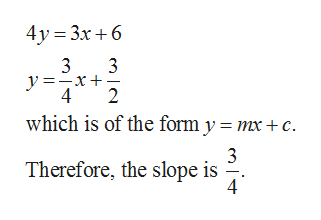# Write an equation of the line that passes through (1,-5) and is parallel to the line define by 3x=4y-6. Write the answer in slope-intercept form (if possible) and in standard form (Ax+By=C) with smallest integer coefficients.

Question
1 views

Write an equation of the line that passes through (1,-5) and is parallel to the line define by 3x=4y-6. Write the answer in slope-intercept form (if possible) and in standard form (Ax+By=C) with smallest integer coefficients.

check_circle

Step 1

Given:

The required line passes through the point (1, –5) and parallel to the line 3x = 4...help_outlineImage Transcriptionclose4y 3x6 3 y=x+ 4 3 2 which is of the form y = mx+c Therefore, the slope is fullscreen

### Want to see the full answer?

See Solution

#### Want to see this answer and more?

Solutions are written by subject experts who are available 24/7. Questions are typically answered within 1 hour.*

See Solution
*Response times may vary by subject and question.
Tagged in

### Other